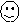#### You may also like### Counting Factors

Is there an efficient way to work out how many factors a large number has?### Summing Consecutive Numbers

15 = 7 + 8 and 10 = 1 + 2 + 3 + 4. Can you say which numbers can be expressed as the sum of two or more consecutive integers?### Helen's Conjecture

Helen made the conjecture that "every multiple of six has more factors than the two numbers either side of it". Is this conjecture true?

# Happy Octopus

##### Age 11 to 14 Challenge Level:Have you met Happy Numbers? Start with any number you like and form a sequence by writing down the sum of the squares of the digits of each number to get the next number in the sequence. For example, from 31 you go to 10 because 3 squared plus 1 squared makes 10. Here are some 'happy' sequences:

31 -> 10 -> 1 -> 1 -> 1 ...68 -> 100 -> 1 -> 1 -> 1 ...25 -> 29 -> 85 -> 89 -> 145 -> 42 -> 20 -> 4 -> 16 -> 37 -> 58 -> 89 ->...

122 -> 9 -> 81 -> 65 -> 61 -> 37 ->...

1122 -> 10 -> 1 -> 1 ;...Numbers are called 'happy' when their sequences, sooner or later, give repeated 1's. We say 1 is a fixed point. The numbers 31, 68 and 1122 are happy and so are the numbers 10 and 100.

The number 25 is sad because, however long we go on with the sequence, it will never come to 1, it will just keep repeating the terms (89, 145, 42, 20, 4, 16, 37, 58) over and over again. We call this an 8-cycle or loop. Can you see why 122 is also a sad number? Can you find some more happy numbers?

This investigation is about happy numbers in the World of the Octopus where all numbers are written in base 8. Octi the octopus counts:

1, 2, 3, 4, 5, 6, 7, 10, 11, 12, 13, 14, 15, 16, 17, 20, 21, 22, 23, 24, 25 ... and so on.

In base 8 the number "one-zero" (written 10) means eight, the number "one-one" means nine etc. and the number "two-zero" (written 20) means two eights or sixteen in base 10. Here are two 'happy' sequences with all numbers written in base 8:

24 -> 24 -> 24 -> 24 -> 24 -> ...

31 -> 12 -> 5-> 31 -> 12 -> 5 ...

We found that twenty, in base 10, that is two-four in base 8, was a sad number. Octi would call two-four a 'happy number' because its sequence 'homes in' on the repeated term (or fixed point) 24.

Three-one, in base 8, is twenty five in base ten; one-two in base 8 is ten in base ten. So, from the sequence above, we can see that three-one, one-two and five are all sad numbers.

Find all the fixed points and cycles for the happy number sequences in base 8.